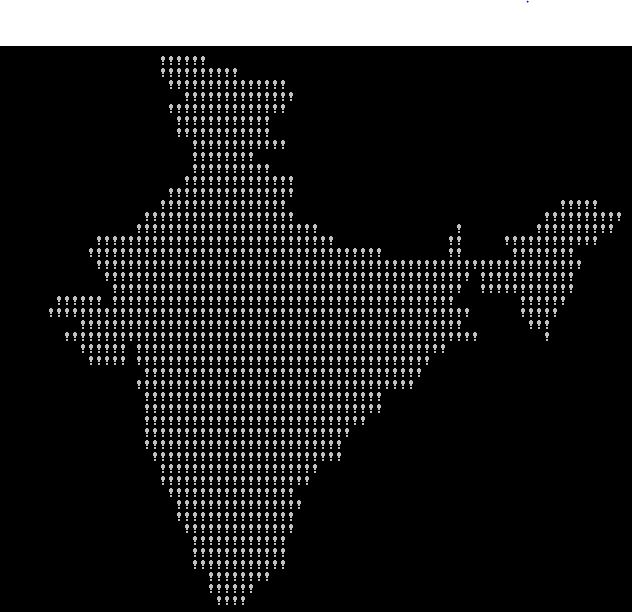Related Articles

# Code to generate the map of India (with explanation)

• Difficulty Level : Expert
• Last Updated : 02 Sep, 2021

Given an obfuscated code that generates the map of India, explain its working.
The following code when executed generates the map of India –

` `

The above code is a typical example of obfuscated code i.e. code that is difficult for humans to understand.
How does it work?
Basically, the string is a run-length encoding of the map of India. Alternating characters in the string store how many times to draw space, and how many times to draw an exclamation mark consecutively.
Here is an analysis of the different elements of this program –
The encoded string

```"Hello!Welcome to GeeksForGeeks."
"TFy!QJu ROo TNn(ROo)SLq SLq ULo+UHs UJq TNn*RPn/QPbEWS_JSWQAIJO^NBELPeHBFHT}TnALVlBL"
"OFAkHFOuFETpHCStHAUFAgcEAelclcn^r^r\\tZvYxXyT|S~Pn SPm SOn TNn ULo0ULo#ULo-WHq!WFs XDt!";```

Notice [b+++21] at the end of the encoded string. As b+++21 is equivalent to (b++ + 21) which will evaluate to 31 (10 + 21), the first 31 characters of this string are ignored and do not contribute to anything. The remaining encoded string contains instructions for drawing the map. The individual characters determine how many spaces or exclamation marks to draw consecutively.
Outer for loop
This loop goes over the characters in the string. Each iteration increases the value of b by one and assigns the next character in the string to a.
Inner for loop
This loop draws individual characters, and a new line whenever it reaches the end of the line. Consider this putchar statement

`putchar(++c=='Z' ? c = c/9 : 33^b&1);`

As ‘Z’ represents number 90 in ASCII, 90/9 will give us 10 which is a newline character. Decimal 33 is ASCII for ‘!’. Toggling the low-order bit of 33 gives you 32, which is ASCII for a space. This causes! to be printed if b is odd, and a blank space to be printed if b is even.
Below is a less obfuscated version of the above code –

## C++

 `// C++ program to print map of India``#include ``using` `namespace` `std;` `int` `main()``{``    ``int` `a = 10, b = 0, c = 10;` `    ``// The encoded string after removing first 31 characters``    ``// Its individual characters determine how many spaces``    ``// or exclamation marks to draw consecutively.``    ``char``* str = ``"TFy!QJu ROo TNn(ROo)SLq SLq ULo+UHs UJq "``                ``"TNn*RPn/QPbEWS_JSWQAIJO^NBELPeHBFHT}TnALVlBL"``                ``"OFAkHFOuFETpHCStHAUFAgcEAelclcn^r^r\\tZvYxXyT|S~Pn SPm "``                ``"SOn TNn ULo0ULo#ULo-WHq!WFs XDt!"``;` `    ``while` `(a != 0)``    ``{``        ``// read each character of encoded string``        ``a = str[b++];``        ``while` `(a-- > 64)``        ``{``            ``if` `(++c == 90) ``// 'Z' is 90 in ascii``            ``{``                ``// reset c to 10 when the end of line is reached``                ``c = 10;        ``// '\n' is 10 in ascii` `                ``// print newline``                ``putchar``(``'\n'``); ``// or putchar(c);``            ``}``            ``else``            ``{``                ``// draw the appropriate character``                ``// depending on whether b is even or odd``                ``if` `(b % 2 == 0)``                    ``putchar``(``'!'``);``                ``else``                    ``putchar``(``' '``);``            ``}``        ``}``    ``}` `    ``return` `0;``}` `// This code is contributed by SHUBHAMSINGH10.`

## C

 `// C program to print map of India``#include ` `int` `main()``{``    ``int` `a = 10, b = 0, c = 10;` `    ``// The encoded string after removing first 31 characters``    ``// Its individual characters determine how many spaces``    ``// or exclamation marks to draw consecutively.``    ``char``* str = ``"TFy!QJu ROo TNn(ROo)SLq SLq ULo+UHs UJq "``                ``"TNn*RPn/QPbEWS_JSWQAIJO^NBELPeHBFHT}TnALVlBL"``                ``"OFAkHFOuFETpHCStHAUFAgcEAelclcn^r^r\\tZvYxXyT|S~Pn SPm "``                ``"SOn TNn ULo0ULo#ULo-WHq!WFs XDt!"``;` `    ``while` `(a != 0)``    ``{``        ``// read each character of encoded string``        ``a = str[b++];``        ``while` `(a-- > 64)``        ``{``            ``if` `(++c == 90) ``// 'Z' is 90 in ascii``            ``{``                ``// reset c to 10 when the end of line is reached``                ``c = 10;        ``// '\n' is 10 in ascii` `                ``// print newline``                ``putchar``(``'\n'``); ``// or putchar(c);``            ``}``            ``else``            ``{``                ``// draw the appropriate character``                ``// depending on whether b is even or odd``                ``if` `(b % 2 == 0)``                    ``putchar``(``'!'``);``                ``else``                    ``putchar``(``' '``);``            ``}``        ``}``    ``}` `    ``return` `0;``}`

## Java

 `// Java program to print map of India``class` `GFG``{``    ``public` `static` `void` `main(String[] args)``    ``{``        ``int` `a =``10``, b = ``0``, c = ``10``;``        ` `        ``// The encoded string after removing first 31 characters``        ``// Its individual characters determine how many spaces``        ``// or exclamation marks to draw consecutively.``        ``String s1=``"TFy!QJu ROo TNn(ROo)SLq SLq ULo+UHs UJq TNn*RPn/QP,\n"``        ``+ ``"bEWS_JSWQAIJO^NBELPeHBFHT}TnALVlBLOFAkHFOuFETpHCStHAUFAgcEAelc,\n"``        ``+ ``"lcn^r^r\\tZvYxXyT|S~Pn SPm SOn TNn ULo0ULo#ULo-WHq!WFs XDt!"``;``        ` `        ``// read each character of encoded string``        ``a=s1.charAt(b);``        ` `        ``while` `(a != ``0``)``        ``{``            ``if` `(b < ``170``)``            ``{``                ``a = s1.charAt(b);``                ``b++;``                ``while` `(a-- > ``64``)``                ``{``                    ` `                    ``if` `(++c==``'Z'``)``                    ``{``                        ` `                        ``c/=``9``;``                        ` `                        ``System.out.print((``char``)(c));``                    ``}``                    ``else``                        ``System.out.print((``char``)(``33` `^ (b & ``0x01``)));``                ``}``            ``}``            ``else``                ``break``;``        ``}``    ``}``}`

## Python3

 `# Python3 program to print map of India``a ``=` `10``b ``=` `0``c ``=` `10` `# The encoded string after removing first``# 31 characters. Its individual characters``# determine how many spaces or exclamation``# marks to draw consecutively.``s ``=` `(``"TFy!QJu ROo TNn(ROo)SLq SLq ULo+UHs"``     ``" UJq TNn*RPn/QPbEWS_JSWQAIJO^NBELPe"``     ``"HBFHT}TnALVlBLOFAkHFOuFETpHCStHAUFA"``     ``"gcEAelclcn^r^r\\tZvYxXyT|S~Pn SPm S"``     ``"On TNn ULo0ULo#ULo-WHq!WFs XDt!"``)` `# Read each character of encoded string``a ``=` `ord``(s[b])` `while` `a !``=` `0``:``    ``if` `b < ``170``:``        ``a ``=` `ord``(s[b])``        ``b ``+``=` `1``        ` `        ``while` `a > ``64``:``            ``a ``-``=` `1``            ``c ``+``=` `1``            ` `            ``if` `c ``=``=` `90``:``                ``c ``=` `c ``/``/` `9``                ``print``(end ``=` `chr``(c))``            ``else``:``                ``print``(``chr``(``33` `^ (b & ``0X01``)), end ``=` `'')``    ``else``:``        ``break` `# The code is contributed by aayush_chouhan`

## C#

 `// C# program to print map of India``using` `System;` `class` `GFG``{``    ``public` `static` `void` `Main()``    ``{``        ``int` `a = 10, b = 0, c = 10;``        ` `        ``// The encoded string after removing first 31 characters``        ``// Its individual characters determine how many spaces``        ``// or exclamation marks to draw consecutively.``        ``string` `s1 = ``"TFy!QJu ROo TNn(ROo)SLq SLq ULo+UHs UJq TNn*RPn/QP,\n"``        ``+ ``"bEWS_JSWQAIJO^NBELPeHBFHT}TnALVlBLOFAkHFOuFETpHCStHAUFAgcEAelc,\n"``        ``+ ``"lcn^r^r\\tZvYxXyT|S~Pn SPm SOn TNn ULo0ULo#ULo-WHq!WFs XDt!"``;``        ` `        ``// read each character of encoded string``        ``a = s1[b];``        ` `        ``while` `(a != 0)``        ``{``            ``if` `(b < 170)``            ``{``                ``a = s1[b];``                ``b++;``                ``while` `(a-- > 64)``                ``{``                    ` `                    ``if` `(++c == ``'Z'``)``                    ``{``                        ` `                        ``c/=9;``                        ` `                    ``Console.Write((``char``)(c));``                    ``}``                    ``else``                    ``Console.Write((``char``)(33 ^ (b & 0x01)));``                ``}``            ``}``            ``else``                ``break``;``        ``}``    ``}``}` `//This code is contributed by vt_m.`

## PHP

 ` 64)``            ``{``                ` `                ``if` `(++``\$c``==90)``                ``{``                    ` `                    ``\$c``=``floor``(``\$c``/9);``                    ` `                    ``echo` `chr``(``\$c``);``                ``}``                ``else``                    ``printf(``chr``(33 ^ (``\$b` `& 0x01)));``            ``}``        ``}``        ``else``            ``break``;``    ``}` `// note: ord() function convert the``// characters into its Ascii value` `//this code is contributed by mits``?>`

Output:Reference: http://stackoverflow.com/questions/3533348/how-does-this-code-generate-the-map-of-india